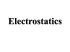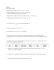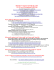# A protein molecule in an electrophoresis gel has a negative charge

## Transcription

A protein molecule in an electrophoresis gel has a negative charge
```A protein molecule in an electrophoresis gel has a negative charge. The exact
charge depends on the pH of the solution, but 30 excess electrons is typical. We
will walk through calculating the magnitude of the electric force on a protein in a
1500 Newtons/Coulomb electric field.
First, we are told to assume that each protein molecule has 30 excess
electrons. We can find the net charge on a protein molecule by multiplying the
number of excess electrons per molecule by the charge of each electron.
We are told in part one of the electricity and magnetism reading that each
electron has a charge of -1.6 x 10-19 Coulombs. Thus, the net charge on a protein
molecule is equal to
(30 electrons/protein molecule) x (-1.6 x 10-19 Coulombs/electron)
= -4.8 x 10-18 Coulombs/protein molecule (Note: I just plugged this into my
calculator to find the answer.)
We are only concerned about the magnitude of the electric force, and so we can
drop the minus sign in front of the charge per protein molecule.
The force by an electric field on a charged particle within the field can be found
with the following formula:
(force on a charged particle) = (the charge of the particle) x (the electric field
strength).
Again, we are only concerned about the magnitude of the force on the protein, and
so we can say:
(the magnitude of the force on the charged particle) = (the magnitude of the
charge of the particle) x (the electric field strength).
Thus, the magnitude of the electric force on a protein particle in the electric field
of strength given above is equal to
(4.8 x 10-18 Coulombs/protein molecule) x (1500 Newtons/Coulomb)
= [y] x 10-15 Newtons. Notes: The magnitude of the charge of the particle comes
from the first part of the calculation, and the electric field strength comes from
the problem statement.
```

### Potential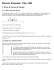### Assignment (02) Introduction to Electric Charges and Electric Field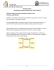### IB Topic 4: Bonding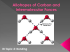### Electrostatics pset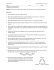### MAGNETISM, n. Something acting upon a magnet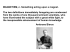### Lewis Structures and the “Real” 3D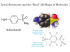### SCH4U1 Investigation: VSEPR Theory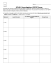### Review